Fluids Index

Hydrostatics

Introduction

Definition (Hydrostatics)...That part of fluid mechanics restricted to fluids in which the velocity (linear or angular) of mass motion does not vary from point to point.   The term hydro comes from a Greek word meaning water.  This term is generally used for water but it also applies to other fluids both liquid and gaseous.

Symbols

 A = Area (m2) Ixx = Second moment of area about horizontal axis (m4) g = acceleration due to gravity (m/s2 ) h = fluid head (m) kG = radius of gyration of surface about centroid (m) kO = radius of gyration of surface about axis O-O'(m) p = fluid pressure (N /m2 ) p s= surface pressure (N /m2 ) p g= gauge pressure (N /m2 ) ρ = fluid density (kg /m2 ) W = weight N x = depth of centroid (m)

Buoyancy

The buoyancy of a body wholly or partly immersed in a fluid at rest , situated in a gravitational field or other field of force is defined as the upward thrust of the fluid on the body.  Generally all problems relating to buoyancy can be resolved by applying the principles of Archimedes.

Put simply
The buoyancy of any body is vectorially equal and opposite to the weight of the fluid displaced by the body and has the same line of action.

The upward thrust which the surrounding fluid exerts on an object is referred to as the force of buoyancy.  This thrust acts through the centroid of the displaced volume, referred to as the centre of buoyancy.  The centre of buoyancy is not the same as the centre of gravity which relates to the distribution of weight within the object.  If the object is a solid with a uniform density exactly the same as water and the body is immersed in water the force of buoyancy will be exactly equal to the weight and the centre of buoyancy will be the same as the centre of gravity. The object will be in equilibrium with the surrounding fluid.

This principle also applied to gases as well as liquids and explains why balloons filled with gases which have lower density compared to air rise to such a height that the weight of the air displaced is equal to the weight of the gas in the balloon.

A body which hovers in a fluid and is in equilibrium is said to have neutral buoyancy.

If the centre of gravity (G) is not in the same location as the centroid (centre of buoyancy-B).  The body will orient itself such that the centre of Gravity is below the centre of buoyancy. (See diagram below). The diagram below shows a hollow vessel with a heavy weight occupying a small segment.  The diagram below shows the object in a fully stable equilibrium position.  In theory if the G was vertically above B then there is no force (moment) tending to rotate the object and it is still in a position of equilibrium.  In this position however it is considered to be unstable.

.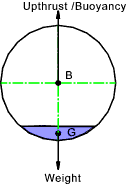The figures below show two positions of a similar submerged object which represent positions of stable and unstable equilibrium.  The definition of stable and unstable equilibrium are stated thus.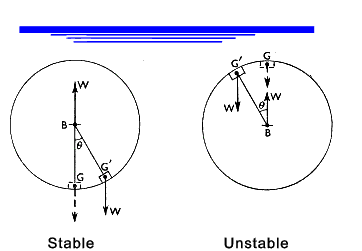If an immersed body initially at rest is displaced so that the force of buoyancy and the force of the centre of gravity are not in the same vertical line :
...The body is stable if the resulting couple tends to bring the body back to its original position and
...The body is unstable if the resulting couple tends to move the body away from its original position.

Metacentre and Metacentric Height

Consider a rectangular vessel immersed as shown below in the first figure the centre of buoyancy at B and the centre of gravity is at G. with the water line at S-S    Now if the vessel is heeled such that the water line is at S'=S'.    The centre of buoyancy now moves to B' as shown in the second figure below.   There is now an upthrust (W) due to buoyancy at B' and the weight of the vessel(W) is acting down at G and there is a couple W.a acting to restore the vessel to its original position.   The locus of each position of B' as the vessel heels to different angles is called the buoyancy curve.  Also the curve joining the tangents of each line of thrust, drawn relative to the vessel, is known as the curve of metacentres.    The cusp of this curve is known as the initial metacentre .  This is shown on the third figure which combines the first and second figures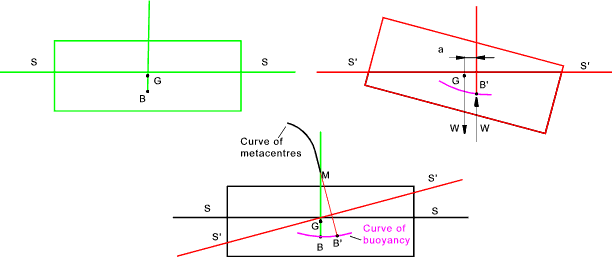The initial metacentre M is the point where the line of action of the upthrust intesects the original vertical line through the centre of buoyancy B and the centre of gravity G for an infinitesimal angle of heel.

The righting moment is calculated as W.GM.sinθ..The angle of heel being θ.   For small values of heel up to about 15o GM is fairly constant and is the value generally accepted as ..the traverse metacentric height of the vessel

A floating vessel is stable if the metacentre lies above the centre of gravity G.
A floating vessel is in neutral equilibrium if the metacentre lies on the centre of gravity G.
A floating vessel is unstable if the metacentre lies below the centre of gravity G.

Pressure in liquids

A perfect fluid cannot resist or exert any shear force and is defined as non viscous or inviscid under all conditions.  The intensity of normal forces is called the pressure and is positive if compressive.  Considering a small element of fluid of uniform thickness which is subject to pressures p, px, and py as shown the element is assumed to be so small that the pressures are assumed to be uniform (the effect of gravity is ignored).  Equating forces in the x and y directions results in the equations

p A sin θ = A sinθ py
p A cos θ = A cosθ px
p = py = px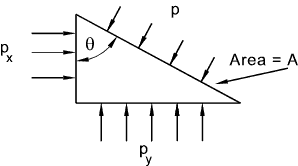This simple example illustrates that for perfect fluids and, to some extent, for real fluids the pressure at a point is the same in all directions.  Thus in static fluids it is reasonable to identify the pressure at a point in any direction of direction.

******
To determine the pressure p at any depth h below a free surface in is necessary to examine the vertical equilibrium of an imaginary vertical cylinder of a fluid.  The fluid column must be supported by the pressure difference across its ends.  If the atmospheric pressure is ps. The weight of the fluid = the density multiplied by the volume ρ.A.h  Assume the cylinder has unit Area A= 1

p - ps = W = ρA.h

The pressure in a liquid under the influence of gravity increases uniformly with depth is proportional to the density and is in addition to the surface pressure.

p = ps + ρgh

Liquids are asssumed to be virtually incompressible and ρ is therefore assumed to be constant.  If the pressure is measured above atmospheric pressure then the pressure is called the gauge pressure pg.    Ordinary dial pressure gauges measure gauge pressure.  The liquid pressure at different depths based on gauge pressure is rewritten as

pg = ρgh

The figure below illustrates the hydrostatic paradox .. It implies that using the relevant formula the force on the inside base of the vessel can be many times the weight of the fluid contained.  This is explained by the fact that most of the downward pressure is balanced by the upward pressure on the downward facing surfaces of the vessel.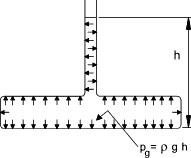Using the pressure at depths to establish the buoyancy consider the figure below.  Assume an immersed body is composed of and infinite number of vertical cylinders each of area δA. and length h.
The upward thrust on each cylinder = ρghδA.   This is equal to ρgv when v = hδA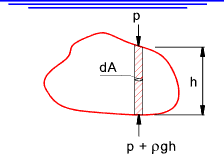.

Adding the upthrust for all the cylinders making up the volume (V) of the immersed body.
V = Σ v and F = the total upthrust (buoyancy).

F = ρg Σ v. = ρg V = The weight of the displaced fluid

If the object is grounded such that the area in contact with the ground is A l there is a loss of buoyancy = ρg hA l
This can have very serious consequences for ships grounded on sandbanks.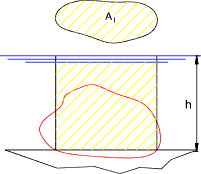.
Force on Submerged surfaces

Consider a submerged plane surface of area A -see figure below.  The surface is subject to a pressure which varies linearly from R to S from pR to pS.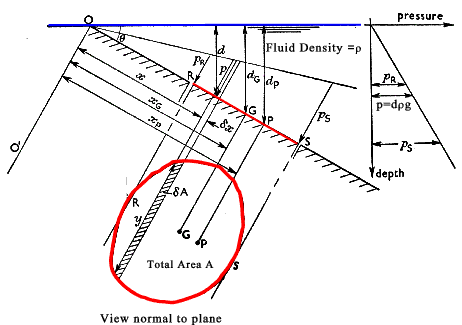The force on each elementary strip =

δF = p.δA = ρgd.δA = ρgx sin θ.δA

The total force =

F = ρg sin θ x .δA

x .δA is the first moment of area of the plane about the line of intersection 0-O' of the immersed surface projected to intersect the liquid surface.   This first moment is equal to A xG where xG is the slant depth of the centroid G.   Now sin θ xG is simply equal to dG which is the depth of the centroid and ρg dG = pG The liquid force acting on the surface is therefore.

F = ρg dGA = pG A

Centre of Pressure on Submerged surfaces

The point at which the resultant fluid force is considered to act on a plane area is called its centre of pressure.   This is shown on the above figure at point P.  This point is found by summing the moments of the elementary forces about the imaginary axis.   O - O'.

M   =   δ M   =     p x δ A   =   ρ  g sin θ  x 2 δ A

This is equivalent to the moment exerted by the resultant force F acting through the centre of pressure P. Thus

M = F xP = [  ρg sin θ  x δA.  ] x P

And from above the force (F) on the plate is

F = ρg sin θ   x .δA

Therefore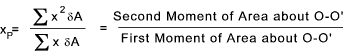The second moment of area of the plane figure about its centroid G is IG
The first moment of area of a plane figure about O - O= A.xG.
Using the parallel axis theorem IO = IG + A. xG2 .
This can be expressed in terms of radii of gyration as A ko2 = A [ kG2 + xG2 ] thereofore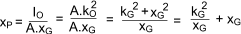Therefore the centre of pressure of a plane area lies below the centroid G of the area by a distance P - G = x P - x G = k G2 / x G measured along the slope of the plane.  As the radius of gyration of the surface about it's centroid k G is fixed the difference reduces as the depth of the surface increases.

 Useful Links Hydrostatics.. (Dr James B. Calvert)..Excellent Document. Hydrostatics..University of Pavia - A very clear guide to the principles - using text, applets, pictures and exercises Basic Hydrostatics..A download document with lots of useful notes equations very well illustrated

Fluids Index